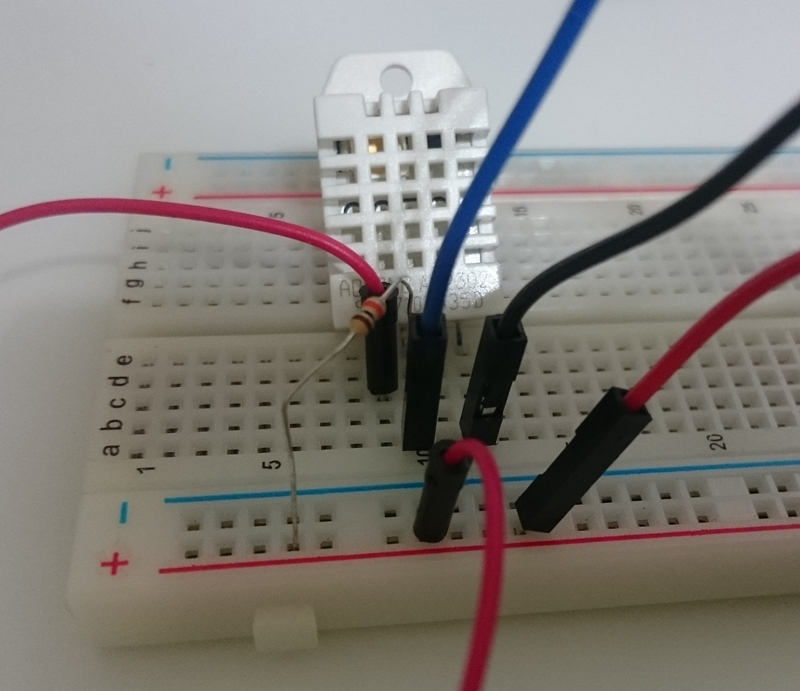# 【NodeMCU】計時器與感測資料的定期量測``````from machine import Pin
import time

ledR = Pin(5, Pin.OUT)
ledB = Pin(4, Pin.OUT)

for i in range(10):
ledR.on()
time.sleep(0.5)
ledR.off()
ledB.on()
time.sleep(0.5)
ledB.off()``````

``````from machine import Pin, Timer

ledR.value(not ledR.value())
ledB.value(not ledB.value())

ledR = Pin(5, Pin.OUT)
ledB = Pin(4, Pin.OUT)

ledR.on()
ledB.off()

t = Timer(0)

``````>>> import dht
>>> from machine import Pin
>>> d = dht.DHT22(Pin(5))
>>> d.measure()
>>> d.temperature()
31.1
>>> d.humidity()
64.8``````

``````from machine import Pin, Timer
import dht

def m(t):
d.measure()
print("{},{}".format(d.temperature(), d.humidity()))

d = dht.DHT22(Pin(5))
t = Timer(0)
t.init(period=50000, mode=Timer.PERIODIC, callback=m)
try:
pass
except:
t.dinit()``````